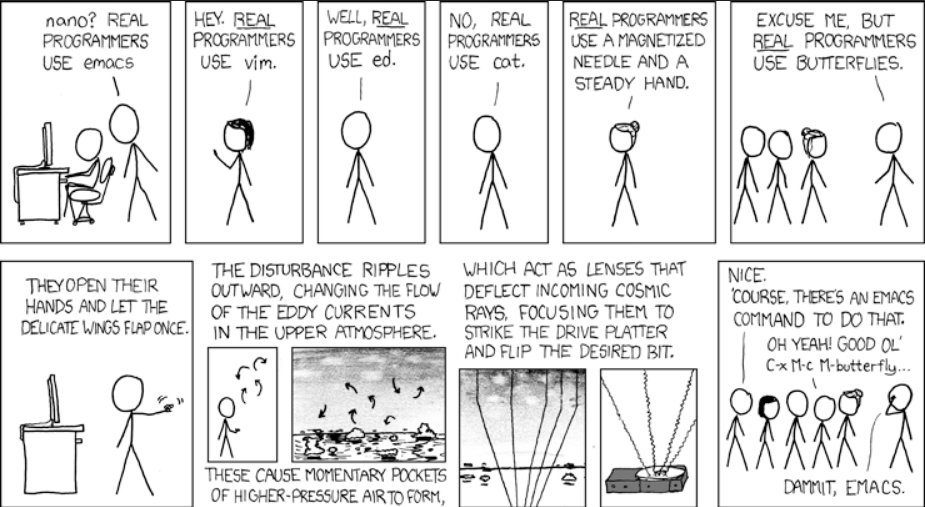# PEP 8 – When to Add Two Blank Lines in Python?

## When to Use Two Blank Lines? PEP 8 Style Guide

The following two rules will provide you a sufficient heuristic on when to use two blank lines:

1. Surround top-level function and class definitions with two blank lines.
2. Insert two blank lines after the import statements if the code that follows starts with a top-level function or class definition.

The second rule is a consequence of the first rule so it could be technically ommitted.

## When to Use Single Blank Lines? PEP 8 Style Guide

The following four rules will provide you a sufficient heuristic on when to use a single blank line:

1. Use one or more extra single blank lines to separate groups of related functions.
2. Use a single blank line in functions to separate logical sections.
3. Use a single blank line to surround method definitions inside a class.
4. Do not use a single blank line between related Python one-liners.

Let’s have a look at some examples in code next!

## Two Blank Lines Top Level Functions

#1.1 – Surround top-level function with two blank lines.

WRONG:

```import x

def f():
pass

f()```

CORRECT:

```import x

def f():
pass

f()```

## One Blank Line Non-Top-Level Function

#1.2 – Surround non-top-level function with single blank lines.

WRONG:

```import x

def f():

def g():
pass

g()

f()```

CORRECT:

```import x

def f():

def g():
pass

g()

f()```

## Two Blank Lines Top-Level Class Definition

#1.3 – Surround top-level class definitions with two blank lines.

WRONG:

```print('hello')

class X:

class Y:
pass

class Z:
pass

print('world')```

CORRECT:

```print('hello')

class X:

class Y:
pass

class Z:
pass

print('world')```

Note that the non-top-level class definitions `Y` and `Z` are not surrounded with two blank lines which is correct and in accordance with the rule.

## Two Blank Lines Import Statements

While many online sources state that there should be two blank lines after the `import` statements before the code starts, this is not generally correct. PEP 8 only states that top-level function or class definitions should be surrounded by two blank lines!

PEP 8 doesn’t talk about `import` statements specifically in regards of insertion of two blank lines!

• If the `import` block is followed by a function or class definition, you should insert two blank lines in accordance to this rule.
• If the `import` block is followed by, say, a global variable definition, you shouldn’t insert two blank lines—one is enough!

Import Statements Followed by Two Blank Lines:

The following code snippet exemplifies the correct insertion of two blank lines after the `import` statement. But the blank lines are not there because of the import statement. They are there because of the top-level function definition of `f`.

```# Correct
import x
import y
import z

def f():
pass

f()```

Import Statements NOT Followed by Two Blank Lines:

The following code snippet exemplifies the correct insertion of only one empty line after the `import` statement because we define a global variable `MY_VAR` that is neither a class nor a function definition and, thereby, shouldn’t be surrounded by two blank lines!

```# Correct
import x
import y
import z

MY_VAR = 42```

The logical implication is that the rule `import` statements should be followed by two blank lines is incorrect!

Next, we’ll explore some examples where only one single blank line can or should be inserted.

## Single Blank Lines

#3 – Use one or more extra single blank lines to separate groups of related functions.

```def f1():
pass

def f2():
pass

def f3():
pass

def g1():
pass

def g2():
pass

def g3():
pass```

#4 – Use a single blank line in functions to separate logical sections.

```def f1():
print('first')
print('logical')
print('section')

print('second')
print('logical')
print('section')

f1()
```

#5 – Use a single blank line to surround method definitions inside a class.

```class X:

def __init__(self):
pass

def x1():
pass

def x2():
pass

def x3():
pass

x = X()

x.x1()
x.x2()
x.x3()
```

A common style error is to surround method definitions by two lines instead of one because people wrongly remember rule #1.

Here’s such a wrong example:

```# WRONG
class X:

def __init__(self):
pass

def x1():
pass

def x2():
pass

def x3():
pass

x = X()

x.x1()
x.x2()
x.x3()
```

Too much whitespace!

Do not surround method definitions with two blank lines!

## Blank Lines Around One-Liners

#6 – Do not use a single blank line between related Python one-liners.

For example, if you write the specification of three functions for later implementation, you can simply omit the blank lines around one-liner function definitions to avoid too much whitespace in the code.

Like so:

```def f1(): pass
def f2(): pass
def f3(): pass```

## Expected 2 blank lines, found 0 (E302)

Python may raise an error or informational message:

• `expected 2 blank lines, found 0 (E302)`
• `expected 2 blank lines, found 1 (E302)`

To fix this error, surround the top-level function or class definitions with two blank lines instead of zero or one to adhere to the PEP 8 style guide.

### No! Do NOT Do This:

```def f1():
pass
def f2():
pass```

```def f1():
pass

def f2():
pass```

Here are some quick references for further reading.

References:

## Programmer Humor“Real programmers set the universal constants at the start such that the universe evolves to contain the disk with the data they want.” — xkcd